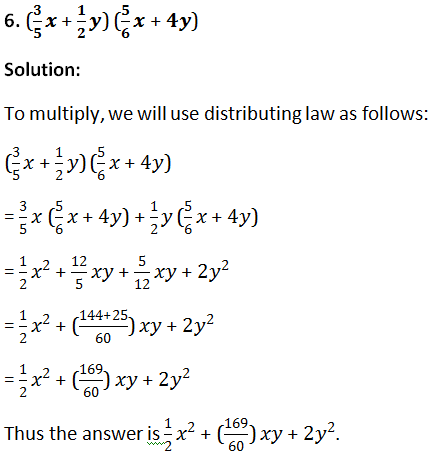# Exercise 6.5

## Multiply:

### 1. (5x + 3) by (7x + 2)

#### Solution:

To multiply, we will use distributive law as follows:

(5x+3)(7x+2)

=5x(7x+2)+3(7x+2)

=(5x×7x+5x×2)+(3×7x+3×2)

=(35x2+10x)+(21x+6)

=35x2+10x+21x+6

=35x2+31x+6

### 2. (2x + 8) by (x – 3)

#### Solution:

To multiply, we will use distributive law as follows:

(2x+8)  (x–3)

=  2x(x–3) +8 (x–3)

= (2x × x–2x × 3) + (8x–8×3)

= (2x2–6x) + (8x–24)

= 2x2–6x + 8x–24 = 2x2+2x–24

### 3. (7x + y) by (x + 5y)

#### Solution:

To multiply, we will use distributive law as follows:

(7x+y)(x+5y)

=7x(x+5y)+y(x+5y)

=7x2+35xy+xy+5y2

=7x2+36xy+5y2

### 4. (a – 1) by (0.1a2 + 3)

#### Solution:

To multiply, we will use distributive law as follows:

(a–1)(0.1a2+3)

=0.1a2(a–1)+3(a–1)

=0.1a3–0.1a2+3a–3

### 5. (3x2+y2)(2x2+3y2)

#### Solution:

To multiply, we will use distributive law as follows:

(3x2+y2)(2x2+3y2)

=3x2(2x2+3y2)+y2(2x2+3y2)

=6x4+9x2y2+2x2y2+3y4

=6x4+11x2y2+3y4### 7. (x6–y6) (x2+y2)

Solution

To multiply, we will use distributive law as follows:

(x6–y6) (x2+y2)

=x6(x2+y2) – y6(x2+y2)

=(x8+x6y2) – (y6x2+y8)

=x8+x6y2–y6x2–y8

### 8. (x2+y2) (3a+2b)

#### Solution:

To multiply, we will use distributive law as follows:

(x2+y2) (3a+2b)

=x2(3a+2b)+y2(3a+2b)

=3ax2+2bx2+3ay2+2by2

### 9. [–3d+(–7f)] (5d+f)

#### Solution:

To multiply, we will use distributive law as follows:

[–3d+(–7f)](5d+f)

= (–3d)(5d+f) + (–7f)(5d+f)

= (–15d2–3df) + (–35df–7f2)

= –15d2–3df–35df–7f2

= –15d2–38df–7f2

### 10. (0.8a–0.5b) (1.5a–3b)

#### Solution:

To multiply, we will use distributive law as follows:

(0.8a–0.5b) (1.5a–3b)

= 0.8a (1.5a–3b) – 0.5b (1.5a–3b)

= 1.2a2–2.4ab –0.75ab+1.5b2

= 1.2a2–3.15ab+1.5b2

### 11. (2x2y2–5xy2)(x2–y2)

#### Solution:

To multiply, we will use distributive law as follows:

(2x2y2–5xy2) (x2–y2)

= 2x2y2 (x2–y2)–5xy2 (x2–y2)

= 2x4y2–2x2y4–5x3y2+5xy4### 14. (3x2y–5xy2)(15x2+13y2)

#### Solution:

To multiply, we will use distributive law as follows:

(3x2y–5xy2)(15x2+13y2)

=15x2(3x2y–5xy2)+13y2(3x2y–5xy2)

=35x4y–x3y2+x2y3–53xy4

### 15. (2x2–1) (4x3+5x2)

#### Solution:

To multiply, we will use distributive law as follows:

(2x2–1) (4x3+5x2)

=2x2(4x3+5x2)–1(4x3+5x2)

=8x5+10x4–4x3–5x2

### 16. (2xy+3y2) (3y2–2)

#### Solution:

To multiply, we will use distributive law as follows:

(2xy+3y2)(3y2–2)

=2xy(3y2–2)+3y2(3y2–2)

=6xy3–4xy+9y4–6y2

=9y4+6xy3–6y2–4xy

## Find the following products and verify the result for x = -1 and y = -2:

### 17. (3x–5y) (x+y)

#### Solution:

To multiply, we will use distributive law as follows:

(3x–5y)(x+y)

= 3x(x+y)–5y(x+y)

= 3x2+3xy–5xy–5y2

=3x2–2xy –5y2

= ∴(3x–5y) (x+y)

=  3x2 –2xy –5y2

Now, we put x = -1 and y = -2 on both sides to verify the result.

LHS = (3x–5y) (x+y)

= {3(−1) –5(−2)} {−1+ (−2)}

= (−3+10) (−3)

= −21

RHS = 3x2 – 2xy–5y2

=3(−1)2–2(−1)(−2)–5(−2)2

=3×1–4–5×4

=3–4–20

=−21

Because LHS is equal to RHS, the result is verified.

### 18. (x2y–1) (3–2x2y)

#### Solution:

To multiply, we will use distributive law as follows:

(x2y–1)(3–2x2y)

=x2y(3–2x2y)–1(3–2x2y)

=3x2y–2x4y2–3+22y

=5x2y–2x4y2–3

∴ (x2y–1)(3–2x2y)=5x2y–2x4y2–3

Now, we put x = -1 and y = -2 on both sides to verify the result.

LHS = (x2y–1)(3–2x2y)

= [(−1)2(−2)–1][3–2(−1)2(−2)]

= [1×(−2)–1][3–2×1×(−2)]

= (−2–1)(3+4)

= −3×7

= −21

RHS = 5x2y–2x4y2–3

= 5(−1)2(−2)–2(−1)4(−2)2–3

= [5×1×(−2)]–[2×1×4]–3

= –10–8–3

=−21

Because LHS is equal to RHS, the result is verified.

## Simplify:

### 20. x2(x+2y) (x–3y)

#### Solution:

To simplify, we will use distributive law as follows:

x2 (x+2y)(x–3y)

=[x2(x+2y)] (x–3y)

=(x3+2x2y)(x–3y)

=x3(x–3y) +2x2y(x–3y)

=x4–3x3+2x3–6x2y2

=x4–x3–6x2y2

### 21. (x2–2y2)(x+4y)x2y2

Solution:

To simplify, we will use distributive law as follows:

(x2–2y2)(x+4y)x2y2

=[x2(x+4y)–2y2(x+4y)]x2y2

=(x3+4x2y–2xy2–8y3)x2y2

=x5y2+4x4y3–2x3y4–8x2y5

### 22. a2b2 (a+2b)(3a+b)

#### Solution:

To simplify, we will use distributive law as follows:

a2b2(a+2b) (3a+b)

= [a2b2(a+2b)] (3a+b)

= (a3b2+2a2b3) (3a+b)

= 3a(a3b2+2a2b3)+b(a3b2+2a2b3)

= 3a4b2+6a3b3+a3b3+2a2b4

= 3a4b2+7a3b3+2a2b4

### 23. x2 (x–y)y2 (x+2y)

#### Solution:

To simplify, we will use distributive law as follows:

x2(x–y) y2 (x+2y)

= [x2(x–y)] [y2(x+2y)]

= (x3–x2y) (xy2+2y3)

= x3(xy2+2y3)–x2y(xy2+2y3)

= x4y2+2x3y3–x3y3–2x2y4

= x4y2+x3y3–2x2y4

### 24. (x3–2x2+5x–7)(2x–3)

#### Solution:

To simplify, we will use distributive law as follows:

(x3–2x2+5x–7)(2x–3)

=2x(x3–2x2+5x–7)–3(x3–2x2+5x–7)

=2x4–4x3+10x2–14x–3x3+6x2–15x+21

=2x4–4x3–3x3+10x2+6x2–14x–15x+21

=2x4–7x3+16x2–29x+21

### 25. 2x4–7x3+16x2–29x+21

#### Solution:

To simplify, we will use distributive law as follows:

(5x+3)(x–1)(3x–2)

= [(5x+3)(x–1)](3x–2)

= [5x(x–1)+3(x–1)](3x–2)

= [5x2–5x+3x–3](3x–2)

= 3x(5x2+2x–3)–2(5x2+2x–3)

= 15x3–6x2–9x–[10x2–4x–6]

= 15x3–6x2–9x–10x2+4x+6

= 15x3–16x2–5x+6

Thus, the answer is = 15x3–6x2–9x–10x2+4x+6

= 15x3–16x2–5x+6

### 26. (5–x) (6–5x)(2–x)

#### Solution:

To simplify, we will use distributive law as follows:

(5–x)(6–5x)(2–x)

=[(5–x)(6–5x)](2–x)

=[5(6–5x)–x(6–5x)](2–x)

=(30–25x–6x+5x2)(2–x)

=(30–31x+5x2)(2–x)

=2(30–31x+5x2)–x(30–31x+5×2)

=60–62x+10x2–30x+31x2–5×3

=60–92x+41x2–5×3

### 27. (2x2+3x–5)(3x2–5x+4)

#### Solution:

To simplify, we will use distributive law as follows:

(2x2+3x–5)(3×2–5x+4)

=2x2(3x2–5x+4)+3x(3x2–5x+4)–5(3x2–5x+4)

=6x4–10x3+8x2+9x3–15x2+12x–15x2+25x–20

=6x4–10x3+9x3+8x2–15x2–15x2+25x+12x–20

=6x4–x3–22x2+36x–20

### 28. (3x–2) (2x–3)+(5x–3) (x+1)

#### Solution:

To simplify, we will use distributive law as follows:

(3x–2)(2x–3) + (5x–3)(x+1)

= [(3x–2) (2x–3)] + [(5x–3) (x+1)]

= [3x (2x–3)–2(2x–3)] + [5x(x+1)–3(x+1)]

= 6x2–9x–4x+6+5x2+5x–3x–3

= 6x2+5x2–9x–4x+5x–3x–3+6

= 11x2–11x+3

### 29. (5x–3) (x+2)–(2x+5)(4x–3)

#### Solution:

To simplify, we will use distributive law as follows:

(5x–3)(x+2)–(2x+5)(4x–3)

= [(5x–3)(x+2)]–[(2x+5)(4x–3)]

= [5x(x+2)–3(x+2)]–[2x(4x–3)+5(4x–3)]

= 5x2+10x–3x–6+8x2+6x–20x+15

= 5x2–8x2+10x–3x+6x−20x–6+15

= −3x2–7x+9

### 30. (3x+2y)(4x+3y)–(2x–y)(7x–3y)

#### Solution:

To simplify, we will use distributive law as follows:

(3x+2y)(4x+3y)–(2x–y)(7x–3y)

=[(3x+2y)(4x+3y)]–[(2x–y)(7x–3y)]

=[3x(4x+3y)+2y(4x+3y)]–[2x(7x–3y)–y(7x–3y)]

=12x2+9xy+8xy+6y2–14x2+6xy+7xy–3y2

=12x2–14x2+9xy+8xy+6xy+7xy+6y2–3y2

=–2x2+30xy+3y2

### 31. (x2–3x+2) (5x–2)–(3x2+4x–5)(2x–1)

#### Solution:

To simplify, we will use distributive law as follows:

(x2–3x+2)(5x–2)–(3×2+4x–5)(2x–1)

= [(x2–3x+2)(5x–2)]–[(3×2+4x–5)(2x–1)]

= [5x(x2–3x+2)–2(x2–3x+2)]–[2x(3x2+4x–5)–1(3x2+4x–5)]

= [5x3–15x2+10x–2x2+6x–4]–[6x3+8x2–10x–3x2–4x+5]

= 5x3–15x2+10x–2x2+6x–4–6x3–8x2+10x+3x2+4x–5

= –x3–22x2+30x–9

### 32. (x3–2x2+3x–4)(x–1)–(2x–3)(x2–x+1)

#### Solution:

To simplify, we will use distributive law as follows:

(x3–2x2+3x–4)(x–1)–(2x–3)(x2–x+1)

=[(x3–2x2+3x–4)(x–1)]–[(2x–3)(x2–x+1)]

=[x(x3–2x2+3x–4)–1(x3–2x2+3x–4)]–[2x(x2–x+1)–3(x2–x+1)]

=x4–2x3+3x2–4x–x3+2x2–3x+4–2x3+2x2–2x+3x2–3x+3

=x4–2x3–2×3–x3+3x2+2x2+2x2+3x2–4x–3x–2x–3x+4+3

=x4–5×3+10x2–12x+7